# How to draw 2.6 million polygons on Android at 60 FPS: The Optimizations

2020, Sep 06

In last article we were able to render L3 datasets with 20 FPS, however, we could not even see the L2 dataset on screen. In this article, I will be throwing light at some optimizations which can help us achieve our final goal i.e. render full L2 dataset at 60FPS.

This post is part 3 of 3 part article series.

Full working sample for this article series can be found on Github

There can be 2 types of optimizations that we can do here

1. Reduce the amount of data that needs to be transferred to GPU for rendering.
2. Employ some OpenGL techniques to do this transfer in an efficient manner.

Let’s begin

## Reduce the amount of Data

Currently, we are rendering data in Immediate Mode, which essentially means that complete `FloatBuffer` is being passed to GPU per frame. That’s a lot of data per second.

### Ditch Z coordinate

In our case, the Z coordinate is always constant its always 0.0. We can just remove this from `FloatBuffer` and move to the shader. Here is the shader that we will be using now

``````// A constant representing the combined model/view/projection matrix.
uniform mat4 u_MVPMatrix;

// Per-vertex position needs only 2 elements and hence vec2
attribute vec2 a_Position;
// Per-vertex color information we will pass in.
attribute vec4 a_Color;
// This will be passed into the fragment shader.
varying vec4 v_Color;

// The entry point for our vertex shader.
void main(){
// Pass the color through to the fragment shader.
// It will be interpolated across the triangle.
v_Color = a_Color;

// gl_Position is a special variable used to store the final position.
// Multiply the vertex by the matrix to get the final point in
// normalized screen coordinates.
// we hardcode z value to 0 here
gl_Position = u_MVPMatrix * vec4(a_Position, 0.0, 1.0);
}
``````

Now position data takes only 2 float values as compared to 3 before. Here is a complete change set for this. This simple optimization alone gave an increase of 10 FPS (50% improvement) on my Google Pixel 3. Now FPS is capped at 30.

### Compute color on GPU- Use lookup table

If you notice we are only using a finite set of colors. In current implementation `getColor()` only returns eight discrete colors. Color can also be computed on GPU using a lookup table.

We can send this lookup table once every frame. So if we have total 8 colors then only 8 RGB values need to be sent to GPU. This is done via `uniform` shader variables. While a vertex shader is executed once for every vertex uniform variable only needs to be sent once for one frame, no matter how many vertices do we have.

Now instead of 3 RGB values per vertex, we send 1 reflectivity value per-vertex and 1 lookup table per frame to the shader. `Reflectivity` value will be used to lookup color from the table. Thus obtained color will be then assigned to the vertex currently being processed. Each vertex now has only 3 elements in `FloatBuffer` that are `X, Y` coordinates, and `Reflectivity` value. This is a 50% reduction in data since we were sending 6 elements per vertex before.

``````vec3 color = vec3(0.0);

// look up color value from table
if(int(a_Reflectivity) < u_colorMap.length()) {
color = u_colorMap[int(a_Reflectivity)];
}

// Pass the color through to the fragment shader.
v_Color = vec4(color, 1.0);

// gl_Position is a special variable used to store the final position.
// Multiply the vertex by the matrix to get the final point in
// normalized screen coordinates.
gl_Position = u_MVPMatrix * vec4(a_Position, 1.0, 1.0);
``````

With this, I also took the liberty to use ~80 colors instead of 8 and use a better-looking lookup table.

Here is a complete set of changes for this optimization. After this L3 dataset is now rendering at full 60 FPS, also, the L2 dataset is successfully rendering at 8 FPS, Finally Yay!!

Here are the results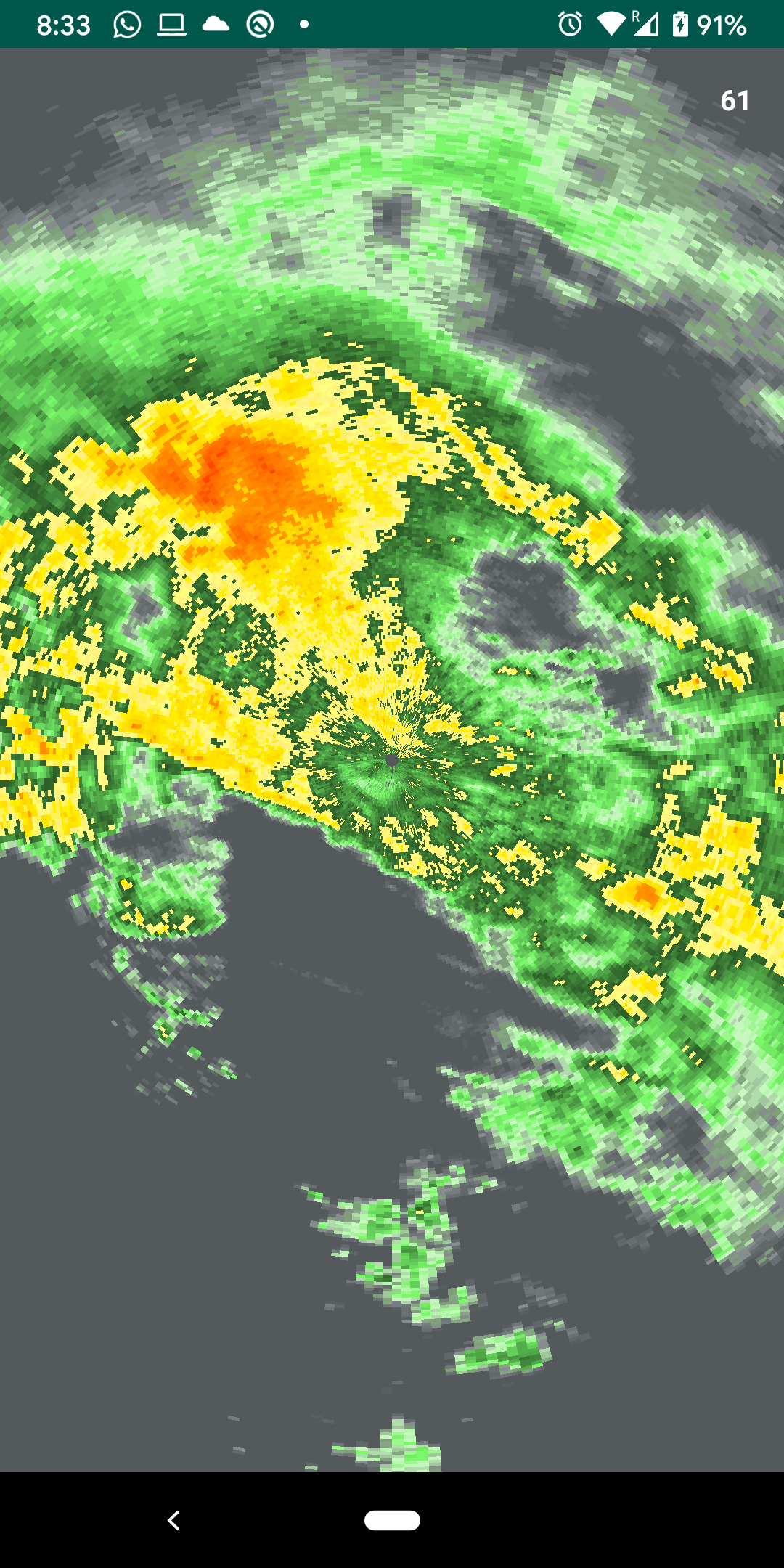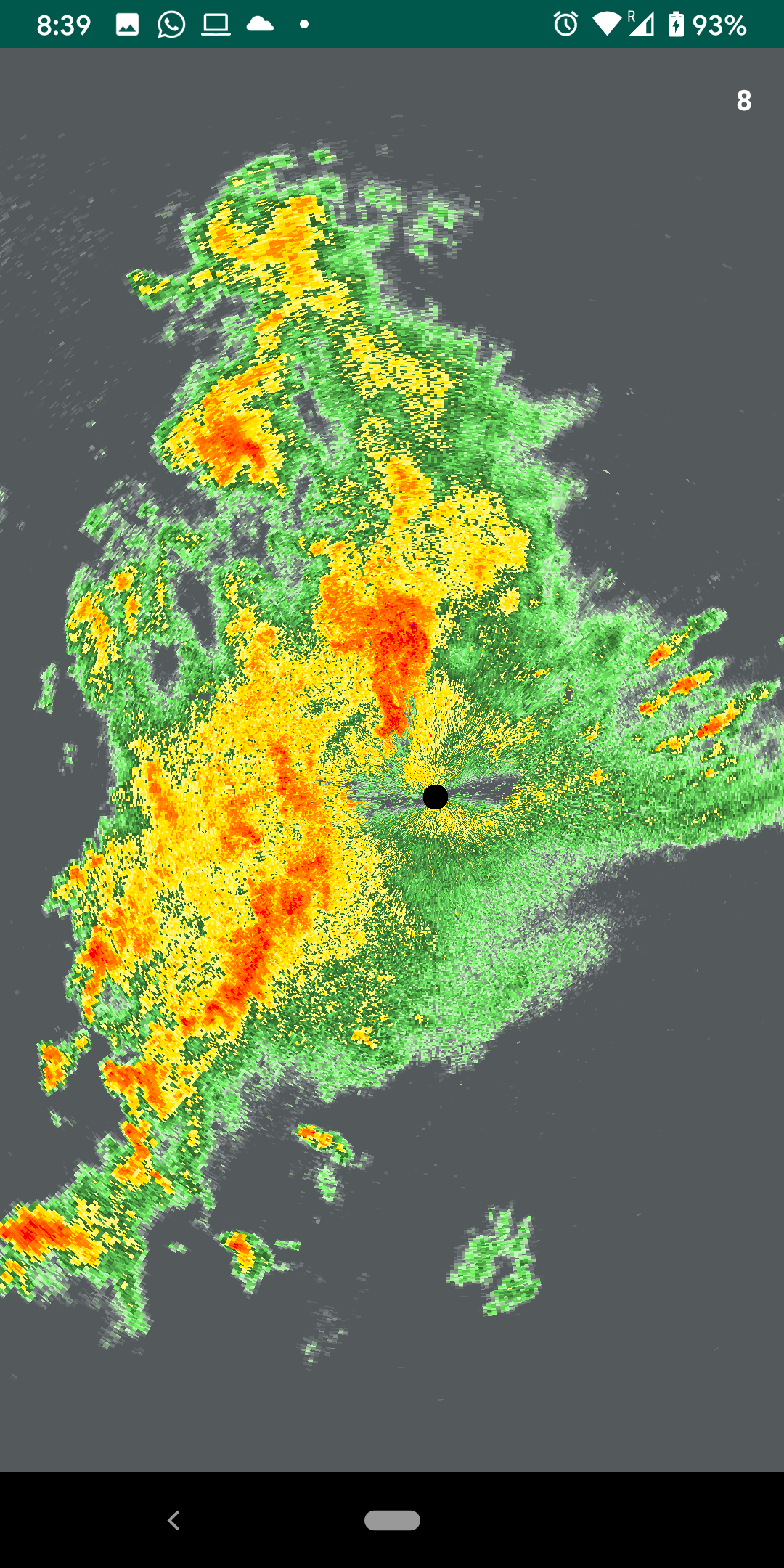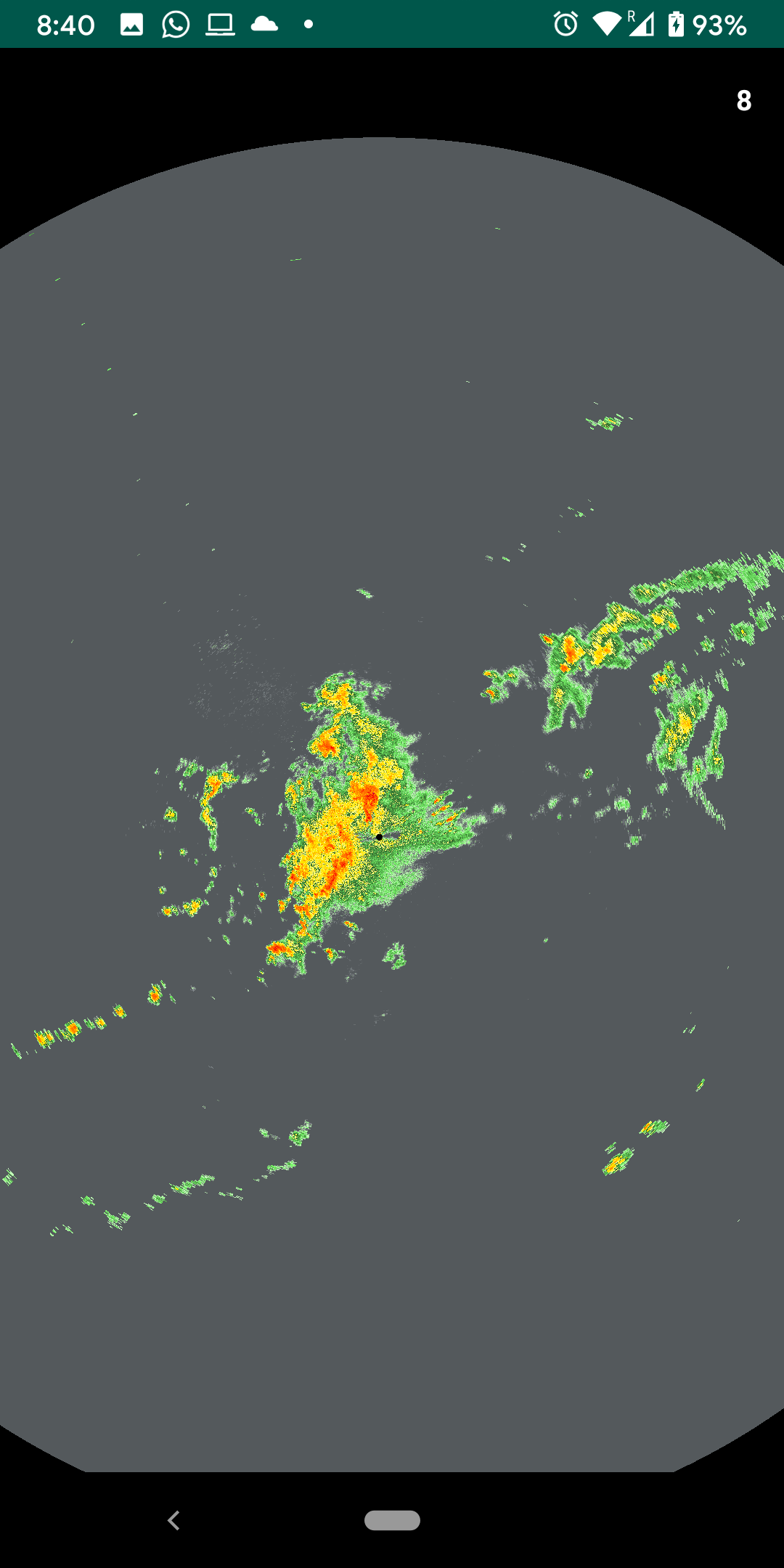Since L3 is already rendering at 60FPS we will work with the L2 dataset from this point onwards.

### Reduce Polygon count

Observe that in the images above there is a lot of empty gray color. This gray color is actually made of polygons that do not have any weather activity or out of range reflectivity values. It’s a waste of effort, memory, and CPU cycles to store these polygons in an array and then transfer to GPU. We can get a little clever and not build polygons when the reflectivity value is less than or zero. We can do this because we only assign one value per trapezoid.

Its pretty easy to implement all we need to do is add `if (reflectivity <= 0) continue` in inner loop of `generateVertexData()` method and when both loops are done set `meshSize = index`. So that we have correct `meshSize` to work within `draw()` method. Depending upon the dataset this is a big win. With the current data set while we had ~23.72mn elements in the array after last optimizations, this alone, brought down the number of elements to ~3.77mn. This is over 6 fold reduction in the number of elements. Since one triangle consists of 9 elements (3 per vertex) this brings down poly count to 418k.

This was the last reasonable optimization in terms of data reduction that I could think of. There are other optimizations like merging polygons with similar reflectivity values but that requires too much preprocessing and not worth it if wanna show real-time visualizations. Also, it forces you to redraw the entire mesh if you go for animations over time.

Also, the L2 dataset is now rendering at around 40FPS on my Google Pixel 3. Here are before and after renders of mesh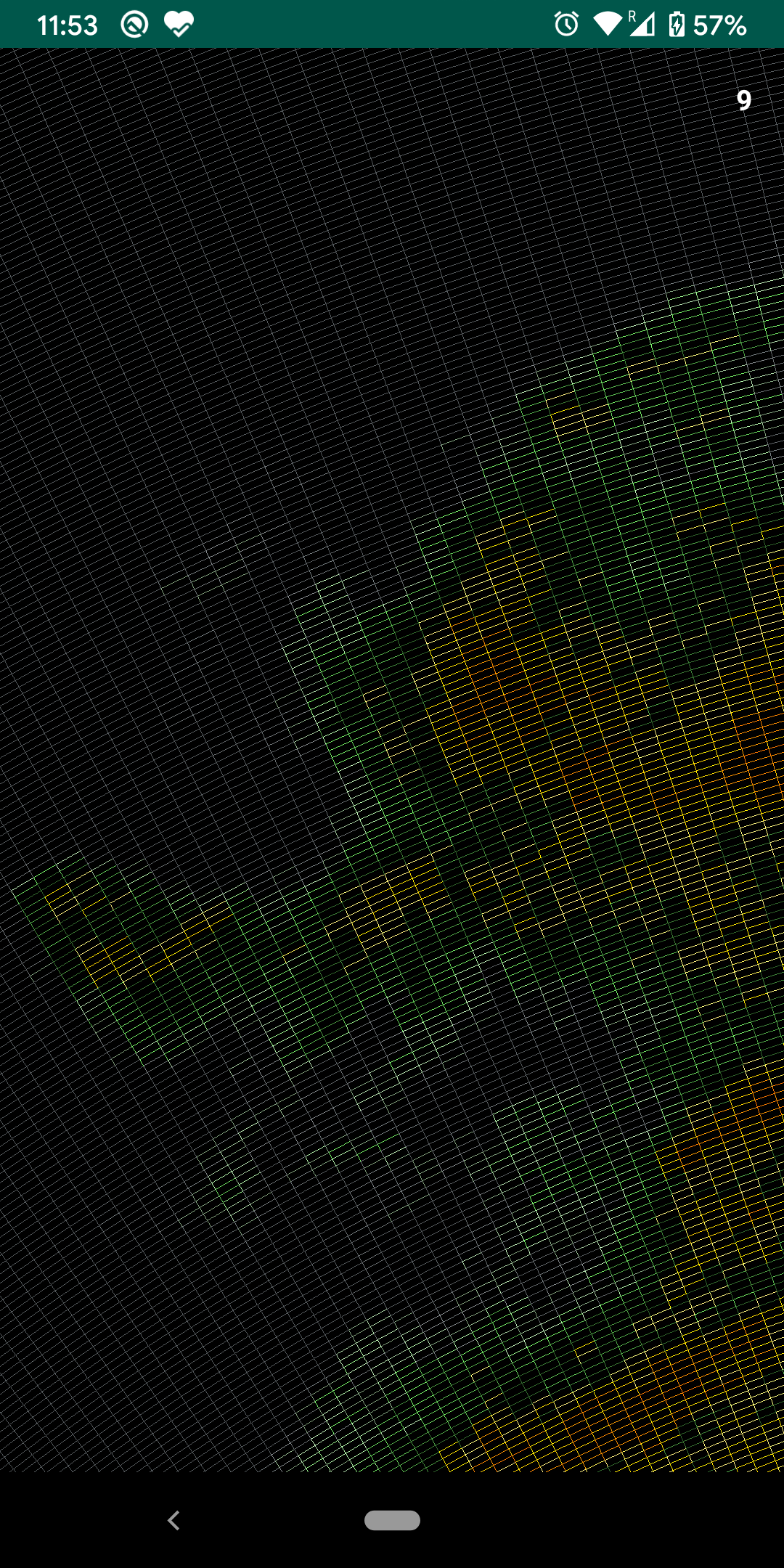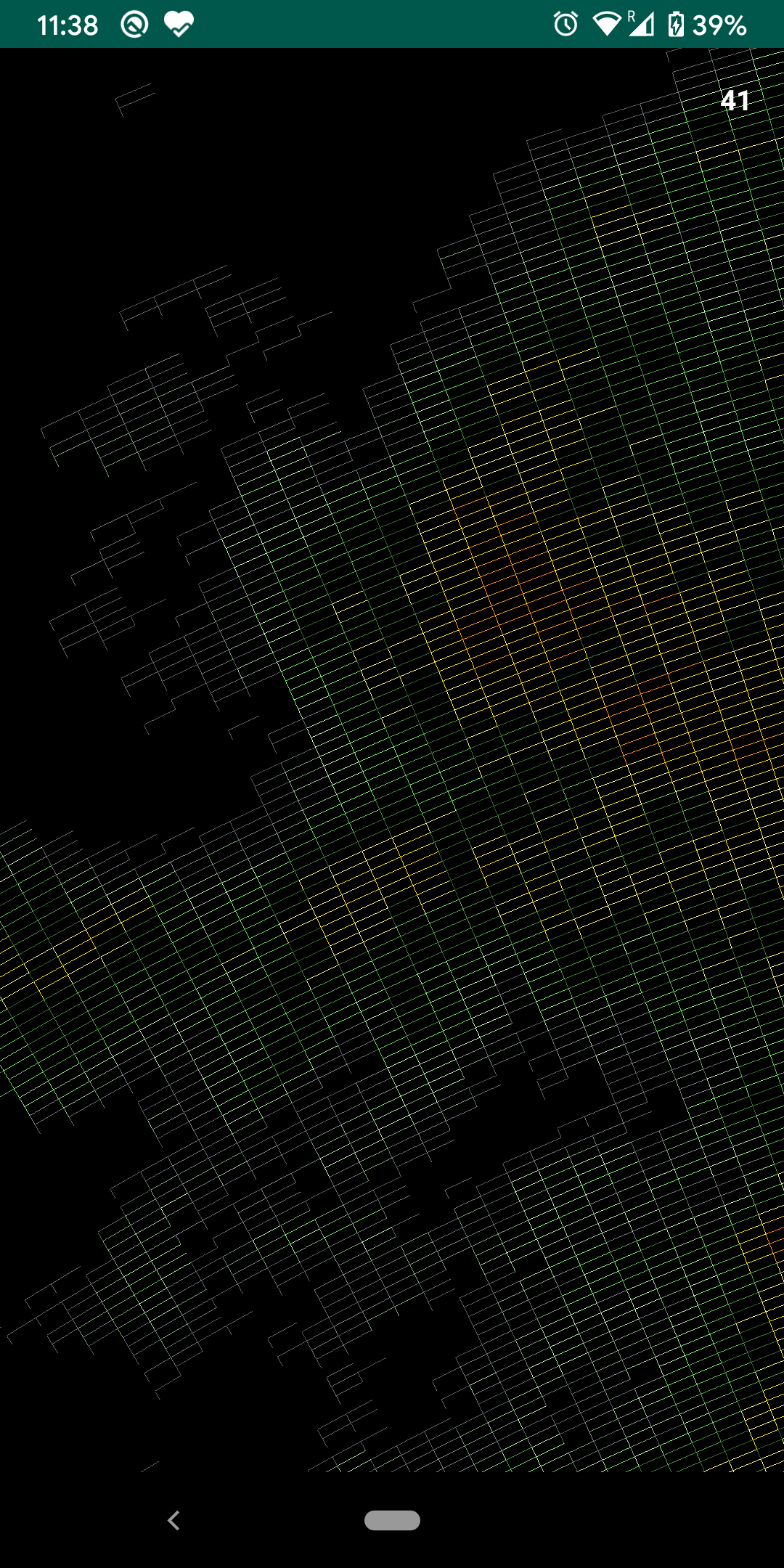Here is a comparison of before and after values to summarize data reduction optimizations

Before After
Triangle Count 8mn 418k
Vertex Count 24mn 1.25mn
Array Element Count 42.8mn 3.77mn

Here is complete changeset for this optimization. Though these are some good numbers our work is not done yet. We still have to hit that sweet 60FPS number. Let now take the help of a great modern OpenGL feature.

## Use VBOs (Vertex Buffer Objects)

I have mentioned this several times that all vertex data that we are generating on CPU needs to be transferred to GPU every frame. This is called `Immediate Mode Rendering`. However, we can instead, generate and transfer data once and save it on GPU memory for subsequent renderings. This is called `Retained Mode` rendering because data is retained in GPU memory.

VertexData transfer between CPU to GPU is a slow process and hence VBOs provide significant performance improvements. Keeping this technique for last is kind of cheating but we would have been wasting so much memory of GPU otherwise. :)

Creating VBO is easy, we just need to transfer our buffer to GPU in advance and obtain a handle to it which we can use later. Here is code that’s doing that

``````// create VBO
// First, generate as many buffers as we need.
// This will give us the OpenGL handles for these buffers.
val buffers = IntArray(1)
GLES30.glGenBuffers(1, buffers, 0)

// Bind to the buffer. Future commands will affect this buffer specifically.
GLES30.glBindBuffer(GLES30.GL_ARRAY_BUFFER, buffers)

// Transfer data from client memory to the buffer.
// We can release the client’s memory after this call.
GLES30.glBufferData(
GLES30.GL_ARRAY_BUFFER, meshSize * mBytesPerFloat,
meshVertices, GLES30.GL_STATIC_DRAW
)

// IMPORTANT: Unbind from the buffer when we're done with it.
GLES30.glBindBuffer(GLES30.GL_ARRAY_BUFFER, 0)
vertexBufferId = buffers
``````

`vertexBufferId` is now an identifier that will be used to ask GPU to use the uploaded buffer. `draw()` method has very few changes and we are ready to roll.

Here is the end result in a GIFAs you can see we have now achieved our end goal of 60 FPS. For the scope of this article series I will stop here, however, there are other OpenGL optimizations that can be applied here like using `Indexed Buffer Objects` or `IBOs`. In that case, instead of duplicating vertices for each triangle, we store indices of those vertices. This will further give some significant savings in terms of GPU memory and CPU utilization at the time of data transfer. I will leave that as an exercise to the reader.

My OpenGL knowledge is pretty limited and hence there can be other optimizations e.g. back-face culling, view frustum culling etc that may or may not be applicable here. Let’s discuss those in the comments.

Happy Coding!